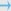number of products in the cart : 0

Quantity

Quantity

Quantity

Quantity

Quantity

Quantity

Quantity

Quantity

Quantity

Quantity

Quantity

Quantity

Quantity

Quantity

Quantity

Quantity

Quantity

Quantity

Quantity

Quantity

Quantity

Quantity

Quantity

Quantity

Quantity

Quantity

Quantity

Quantity

Quantity

Quantity

Quantity

Quantity

Quantity

Quantity

Quantity

Quantity

Quantity

Quantity

Quantity

Quantity

Quantity

Quantity

Quantity

Quantity

Quantity

Quantity

Quantity

Quantity

Quantity

Quantity

Quantity

Quantity

Quantity

Quantity

Quantity

Quantity

Quantity

Quantity

Quantity

Quantity

Quantity

Quantity

Quantity

Quantity

Quantity

Quantity

Quantity

Quantity

Quantity

Quantity

Quantity

Quantity

Quantity

Quantity

Quantity

Quantity

Quantity

Quantity

Quantity

Quantity

Quantity

Quantity

Quantity

Quantity

Quantity

Quantity

Quantity

Quantity

Quantity

Quantity

Quantity

Quantity

Quantity

Quantity

### Production Line

Quantity
 Stay Connected  43 Ha'Azmaut St. P.O.Box 2800 Yehud 56302 Israel Phone: 972-3-6322566 Fax: 972-3-6322644 trust@trust-electronics.com CompanyProfileProductsServicesLine cardTerms Services ListElectronicsTurn KeyRF & MicrowavesRFID KEEP UPDATEDSubscribe to our mailing list for offersnews updates and more!